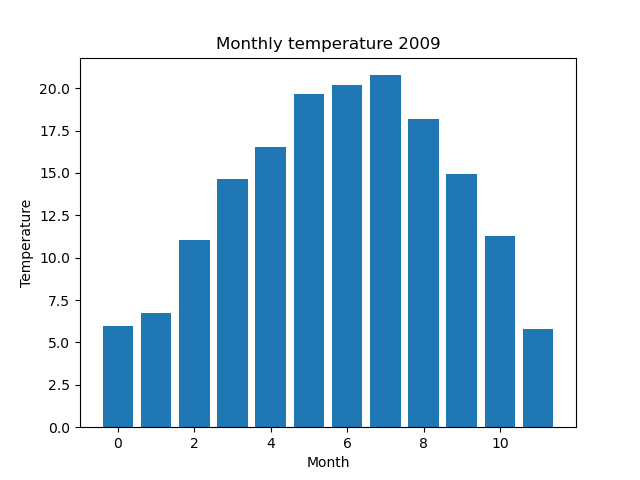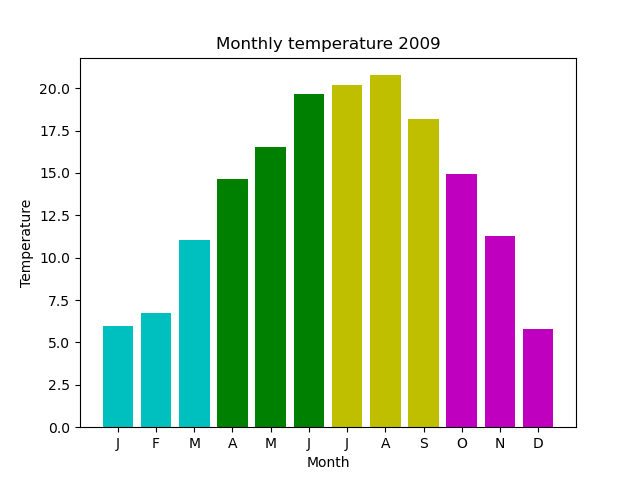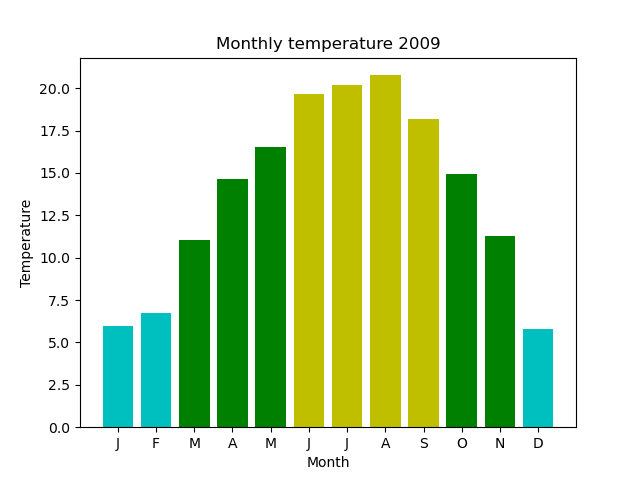# Data colouring Matplotlib bar charts

Martin McBride, 2022-06-23
Tags bar style marker style
Categories matplotlibWe have previously seen how to create a bar chart of the average daily temperatures by month:

We can style the bar chart by controlling the colour and outline of the bar. Here is an example:Matplotlib allows us to set the colour of each bar individually. This chart sets the colour of each month depending on the season:Here is the code to do that:

import matplotlib.pyplot as plt
import csv

with open("2009-temp-monthly.csv") as csv_file:
temperature = [x for x in csv_reader]

month_names = ["J", "F", "M", "A", "M", "J",
"J", "A", "S", "O", "N", "D"]

months = range(12)

plt.title("Monthly temperature 2009")
plt.xlabel("Month")
plt.ylabel("Temperature")
plt.xticks(months, month_names)

colors = ['c', 'c', 'c', 'g', 'g', 'g', 'y', 'y', 'y', 'm', 'm', 'm']

plt.bar(months, temperature, color=colors)
plt.show()


This code is available on github as barchart_monthly_temperatures_seasons.py.

This code is very similar to the previous code for plotting a simple bar chart. The difference is that we have created a list colors, containing 12 color strings (one for each month).

The first three colours are cyan, to represent winter (January, February, and March are predominantly winter). The next three are gree for spring, and so on.

We pass this list into the plt.bar method as the named parameter color. This tells Matplotlib to give each month bar its own colour fron the list. So the first three bars are cyan, the next three are green, etc.

## Making the colours data dependent

So far we have used fixed colours for each month. This effect is mainly decorative, it doesn't tell us a great deal extra about the data.

In this graph we colour each bar according to the temperature value:

• Less than 7.5 degrees is cyan.
• Greater than or equal to 17.5 degrees is yellow.
• The temeperatures in between are green.

Here it is:This is the code:

import matplotlib.pyplot as plt
import csv

with open("2009-temp-monthly.csv") as csv_file:
temperature = [x for x in csv_reader]

month_names = ["J", "F", "M", "A", "M", "J",
"J", "A", "S", "O", "N", "D"]

months = range(12)

plt.title("Monthly temperature 2009")
plt.xlabel("Month")
plt.ylabel("Temperature")
plt.xticks(months, month_names)

colors = ['c' if t < 7.5 else ('g' if t < 17.5 else 'y') for t in temperature]

plt.bar(months, temperature, color=colors)
plt.show()


This code is available on github as barchart_monthly_temperatures_coded.py.

The main work is done by this list comprhension:

colors = ['c' if t < 7.5 else ('g' if t < 17.5 else 'y') for t in temperature]


It loops over the list of 12 monthly temperatures, and sets the colors list to the correct colour based on the value of the temperature.

The code picks the colours dynamically, which means that if you run the same code with different data, the colouring would reflect the new data.

If you found this article useful, you might be interested in the course Introduction to Matplotlib by the same author.

#### Popular tags

2d arrays abstract data type alignment and angle animation arc array arrays bar chart bar style behavioural pattern bezier curve built-in function callable object chain circle classes clipping close closure cmyk colour combinations comparison operator comprehension context context manager conversion count creational pattern data science data types decorator design pattern device space dictionary drawing duck typing efficiency ellipse else encryption enumerate fill filter font font style for loop function function composition function plot functools game development generativepy tutorial generator geometry gif global variable gradient greyscale higher order function hsl html image image processing imagesurface immutable object in operator index inner function input installing iter iterable iterator itertools join l system lambda function len lerp line line plot line style linear gradient linspace list list comprehension logical operator lru_cache magic method mandelbrot mandelbrot set map marker style matplotlib monad mutability named parameter numeric python numpy object open operator optimisation optional parameter or pandas partial application path pattern permutations pie chart polygon positional parameter print pure function python standard library radial gradient range recipes rectangle recursion reduce regular polygon repeat rgb rotation roundrect scaling scatter plot scipy sector segment sequence setup shape singleton slice slicing sound spirograph sprite square str stream string stroke structural pattern subpath symmetric encryption template text text metrics tinkerbell fractal transform translation transparency triangle truthy value tuple turtle unpacking user space vectorisation webserver website while loop zip